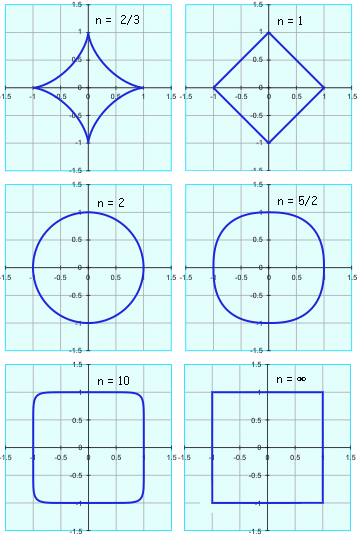All about flooble | fun stuff | Get a free chatterbox | Free JavaScript | Avatarsperplexus dot infoSquare the Circle (Posted on 2004-10-28)There is a family of curves on the Cartesian plane described by this form:If n is equal to 2, then it describes an ellipse (if a = b, then it describes a circle).

If n is greater than 2, then this is a "superellipse" (if a = b, then this is a supercircle).

As n increases, the ellipse becomes more "rectangularish", and as n approaches ∞, the limit is a rectangle (or a square if a=b).

What value must n have such that the figure has an area exactly halfway between the associated ellipse (when n=2) and rectangle (when n=∞)?

The graphs below, calculated by varying n with a = b = 1, show this property. Note that as n approaches zero, the curve degenerates into two crossed lines along the x- and y-axes.No Solution Yet Submitted by SilverKnight Rating: 3.0000 (4 votes)Comments: ( Back to comment list | You must be logged in to post comments.)No Subject| Comment 5 of 8 |In the simplest evaluation a=b=1 we have the area of a circle and the area of a square, which when dealt with in the first quadrant is 1/4 of the area.  Integrating the equation across x:

Integral[(1-x^n)^(1/n) across x from 0 to 1]

When n = 2 (circle) the integral becomes pi/4 or 1/4 the area of a unit circle.

When n = infinity the integral becomes 1 or 1/4 of the area of the associated square (area = 4)

The area for the quarter section half way between would be (1-pi/4)/2 or 0.5-(pi/4)

Now solve the integral (probably numerically) for n such that...
Integral[(1-x^n)^(1/n) across x from 0 to 1] == 0.5-(pi/4)

 Posted by Christian on 2004-10-28 20:37:23Please log in:

 Search: Search body:
Forums (0)